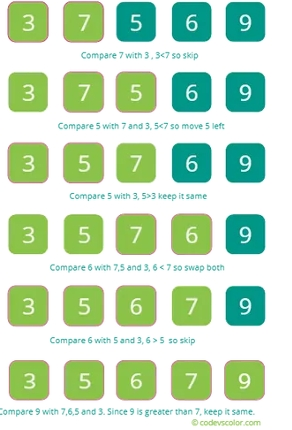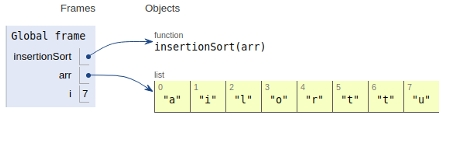# Insertion Sort in Python Program

In this article, we will learn about the implementation of Insertion sort in Python 3.x. Or earlier.

## Algorithm

• Iterate over the input elements by growing the sorted array at each iteration.

• Compare the current element with the largest value available in the sorted array.

• If the current element is greater, then it leaves the element in its place and moves on to the next element else it finds its correct position in the sorted array and moves it to that position in the array.

• This is achieved by shifting all the elements towards the right, which are larger than the current element, in the sorted array to one position ahead.

Now let’s see the visual representation of the algorithmNow let’s see the implementation

## Example

def insertionSort(arr):
for i in range(1, len(arr)):
key = arr[i]
# Move elements of arr[0..i-1], that are greater
than key,
# to one position ahead of their current position
j = i-1
while j >=0 and key < arr[j] :
arr[j+1] = arr[j]
j -= 1
arr[j+1] = key
# main
arr = ['t','u','t','o','r','i','a','l']
insertionSort(arr)
print ("The sorted array is:")
for i in range(len(arr)):
print (arr[i])

## Output

The sorted array is:
a
i
l
o
r
t
t
u

Time Complexity: O(n*2)

Auxiliary Space: O(1)

All the variables are declared in the global frame as shown in the figure below −## Conclusion

In this article, we learnt about the Insertion sort and its implementation in Python 3.x. or earlier.